Courses

# Mathematics Major Test 1

## 31 Questions MCQ Test | Mathematics Major Test 1

Description
This mock test of Mathematics Major Test 1 for Class 11 helps you for every Class 11 entrance exam. This contains 31 Multiple Choice Questions for Class 11 Mathematics Major Test 1 (mcq) to study with solutions a complete question bank. The solved questions answers in this Mathematics Major Test 1 quiz give you a good mix of easy questions and tough questions. Class 11 students definitely take this Mathematics Major Test 1 exercise for a better result in the exam. You can find other Mathematics Major Test 1 extra questions, long questions & short questions for Class 11 on EduRev as well by searching above.
QUESTION: 1

Solution:
QUESTION: 2

Solution:
QUESTION: 3

### The number of the  subset of {a,b,c,} is

Solution:
QUESTION: 4

The set {x :x is an even prime number} can be written as:

Solution:
QUESTION: 5

Let A =  {a,b,} ,B= {a,b,c,} . What is  A∪B:

Solution:
QUESTION: 6

Two finite sets have m and n elements. The total number of subsets of the first set is 56 more than the total number of subsets of the second set. The values of m and n are:

Solution:
QUESTION: 7

In group of 52 persons, 16 drink tea but not coffee, while 33 drink tea. How many persons drink coffee but not tea:

Solution:
QUESTION: 8

There are 600 student in a school. If 400 of them can speak Telugu. 300 can speak Hindi, then the number of students who can speak both Telugu and Hindi is:

Solution:
QUESTION: 9

Value of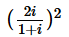is

Solution:
QUESTION: 10

1+i2+i4+i6 is

Solution:
QUESTION: 11

If  (x+iy)(2-3i)=4+i,then

Solution:
QUESTION: 12

if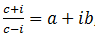where a,b,c are real,then a2+b2 is  equal to :

Solution:
QUESTION: 13

The amplitude of1+i is

Solution:
QUESTION: 14

For z =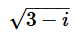, then modulas of z is:

Solution:
QUESTION: 15

if x2+x+1=0,then what is the value of x:

Solution:
QUESTION: 16

The solution of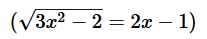are

Solution:
QUESTION: 17

The conjugate of a complex number Is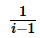then that complex number is

Solution:
QUESTION: 18

The third term of a geometric progression is 4. The product of the first five term is:

Solution:
QUESTION: 19

In an AP. The pth term is q and the (p+q)th term is 0.Then the qth term is:

Solution:
QUESTION: 20

If a,b,c,d,e,f are in A.P.,then e-c is equal to:

Solution:
QUESTION: 21

For a,b,c to be in G.P. what should be the value of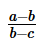Solution:
QUESTION: 22

Find 4th term from the end of the G.P. 3,6,12,24,……..,3072

Solution:
QUESTION: 23

If x + 1, 4x+1, and 8x + 1 are in geometric progression, then what is the non-trivial value of x:

Solution:
QUESTION: 24

Which term of the G.P. 2, 2 √2 ,4,…is 64;

Solution:
QUESTION: 25

Which term of the AP 5,8,11,14,….is 323:

Solution:
QUESTION: 26

(1+3+5+7+…+999)=?

Solution:
QUESTION: 27

The sum of first 80 natural number is:

Solution:
QUESTION: 28

The second and 7th terms of an AP are 2 and 22 respectively. The sum of first 35 terms is:

Solution:
QUESTION: 29

Which term of the GP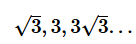is 729?

Solution:
QUESTION: 30

The sum of the infinite series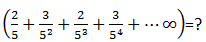Solution:
QUESTION: 31

(12+22+32+...+602) = ?

Solution: